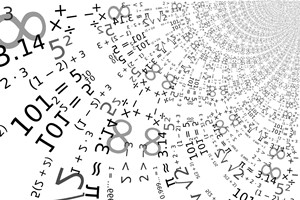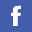Home > Undergraduate > Minor in Statistics (Bulletin 18/19 and after) | (Bulletin 17/18) | (Bulletin 16/17 and before)

Minor in Statistics

Core Courses (6 Units)

MATH2206 Probability and Statistics
or MATH2005 Calculus, Probability, and Statistics for Computer Science
or MATH2006 Calculus, Probability, and Statistics for Science
MATH2207 Linear Algebra

Main Elective Courses (6 Units)

Two courses from the following list:

MATH2216 Statistical Methods and Theory
MATH3805 Regression Analysis
MATH3806 Multivariate Statistical Methods
MATH3816 Statistical Analysis of Sample Surveys

Elective Course (3 Units)

One 3-unit MATHXXXX course, excluding all non-letter grade courses MATH3095-6, MATH3495-7, MATH3097, and MATH4998-9. The GE capstone GCAP3017 can be double-counted towards this 3-unit elective course requirement, and the outstanding 3 units can be made up by taking any 3 units of free elective.

Remarks:

1. No courses may be double counted between a major and a minor programme or between two minor programmes. In such cases, core course(s) can be replaced with elective course(s) to fulfill the unit requirement for this programme.
2. Students of Faculty of Science are not allowed to use MATH1025 Introduction to Mathematics and Statistics to fulfill the Minor requirement. They are required to take another elective course.
3. BSc COMPUTER SCI students are not allowed to use MATH1205 and MATH2005 to fulfill the Minor requirement. They are required to take another elective course.BSc (Hons) in Mathematics and Statistics

The programme is designed to provide students with a solid foundation in mathematical and statistical concepts with an aim towards developing logical thinking and analytical skills.HKBU/SFU Double Degree

The double degree approach leverages the quantitative and market skills of mathematics, statistics and actuarial science with corporate financial management practices.Courses

Major Discipline courses and free elective courses provided by the Department.

Details